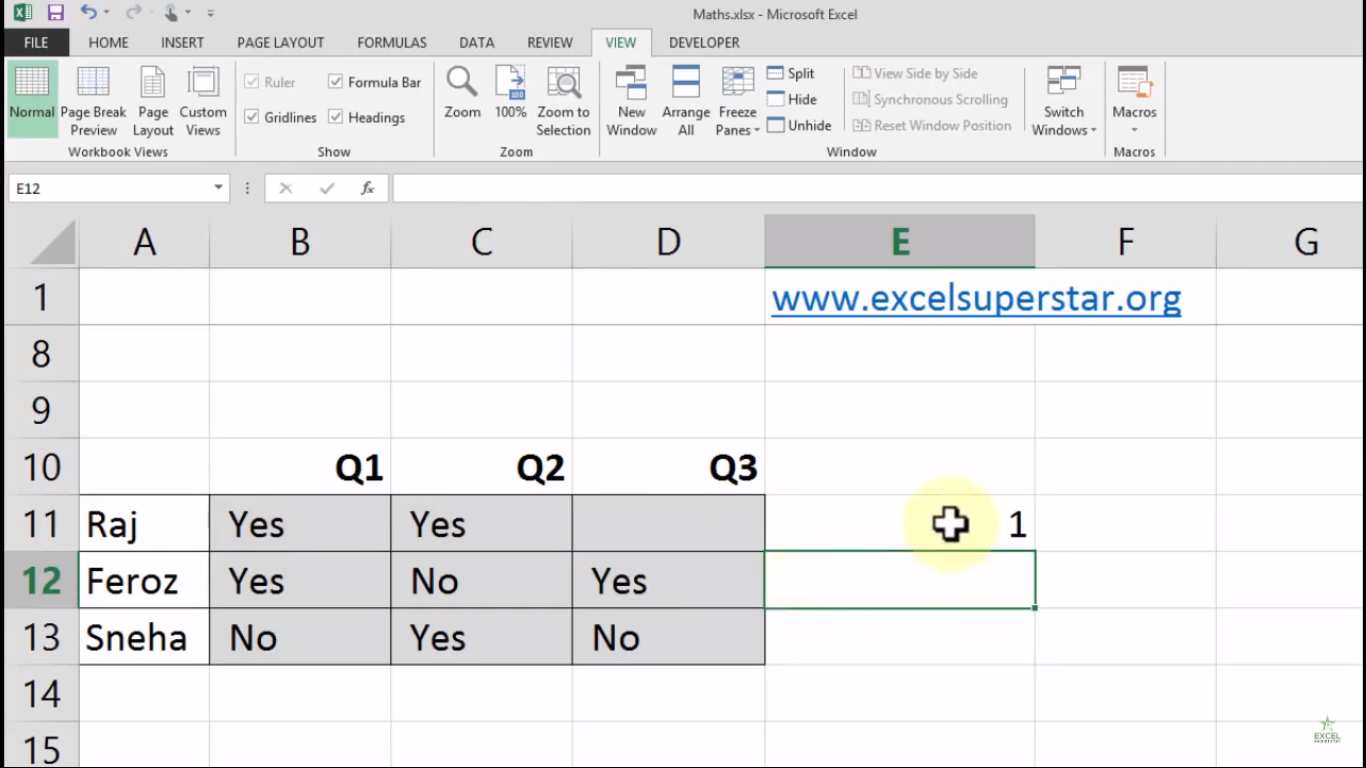# Excel Count Blank FormulaIn this tutorial we will learn about Count Blank Formula in Excel

Case 1:I have a table that consists of 3 columns of different bills along with the name of the persons. Now I want to the number of bills which are blank in the given row

So to count the number of blank cells we will be applying Count Blank Formula by following the steps given below:

1. Write =count and select the option COUNTBLANK by pressing the Tab Key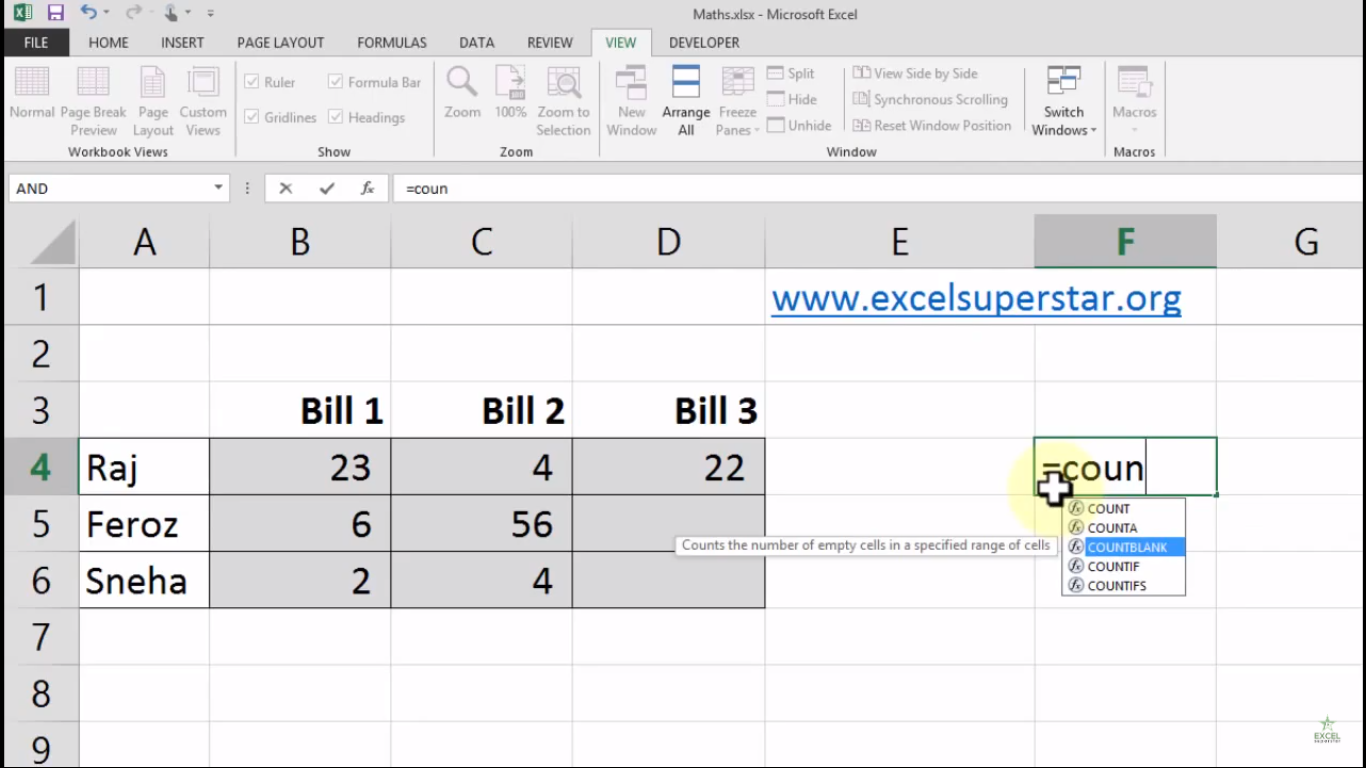2. Select all the grey cells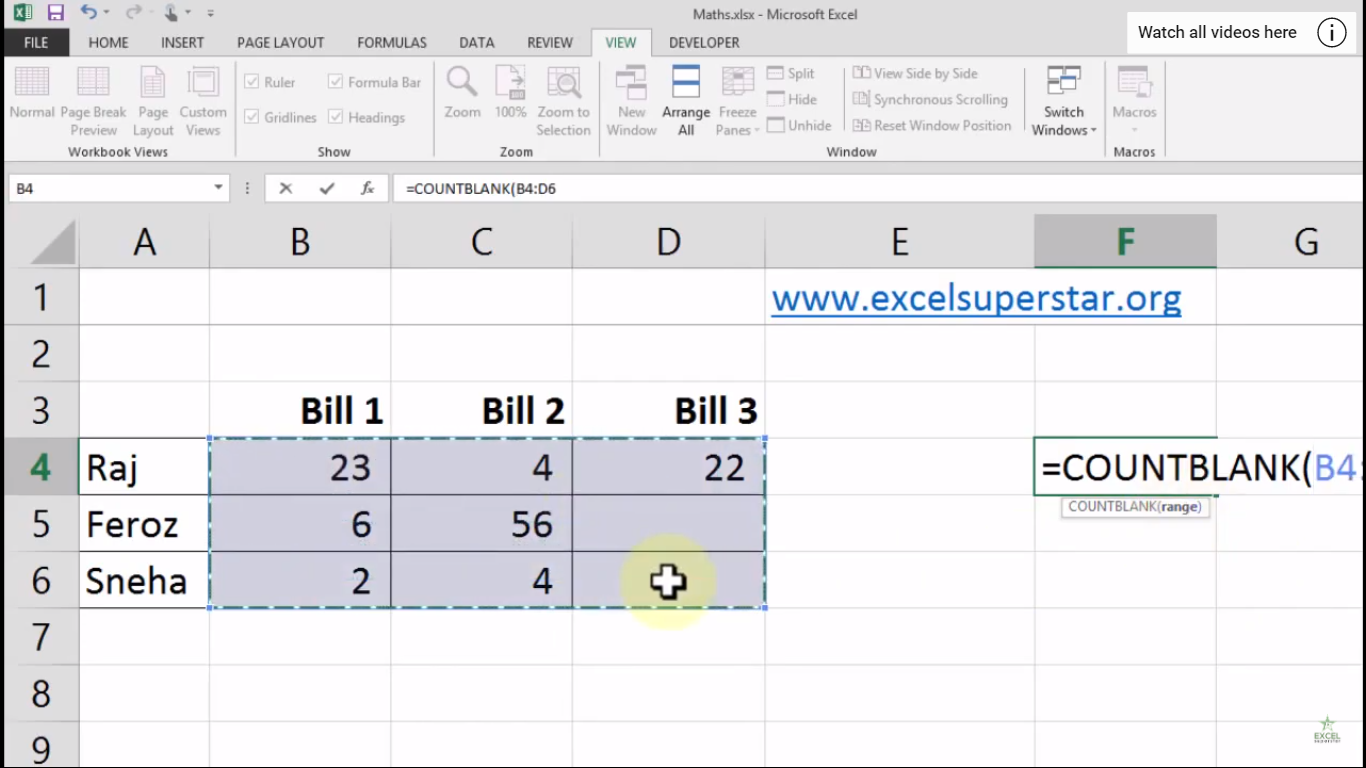3. Close the bracket and press Enter

4. Lastly, you will get the answer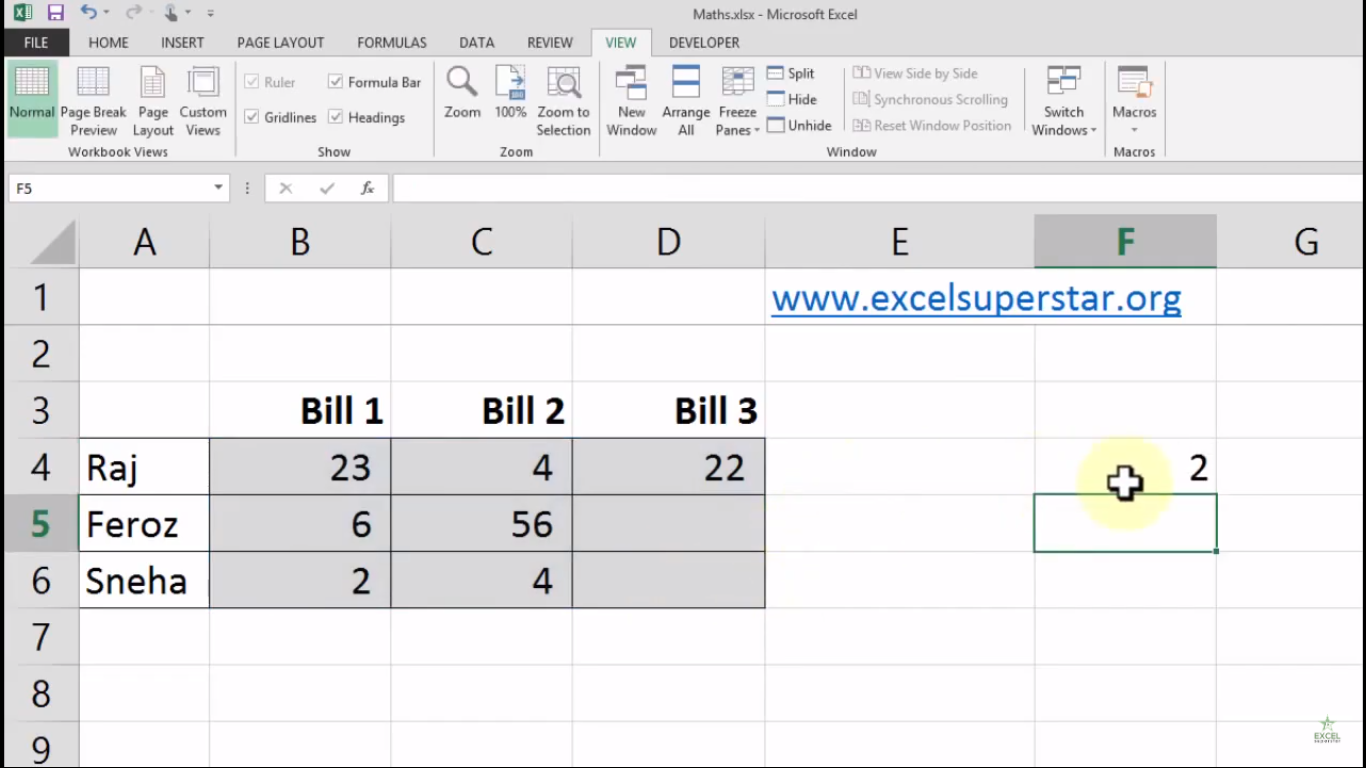Note – If there is a spacebar in a given cell then it will not be considered as blank and because it is blank then Count Blank will not add it up.

Case 2: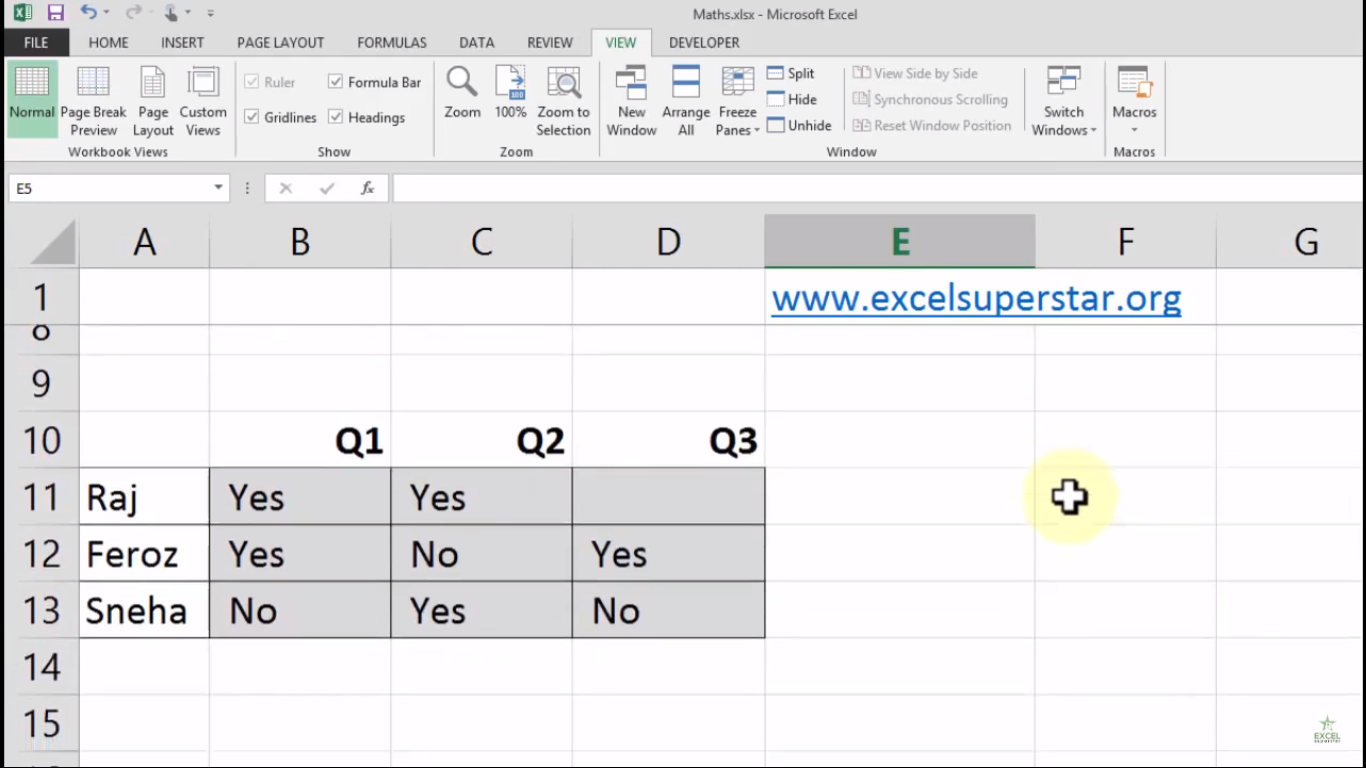In this table, I have asked all the students whether the subject was understandable or not. Many students said Yes or No and some students kept unanswered.

Now I want to know the number of students who kept unanswered. So here we will be applying Count Blank Formula to count the number of cells by following the steps given below:

1. Write =count and select the option COUNTBLANK by pressing the Tab Key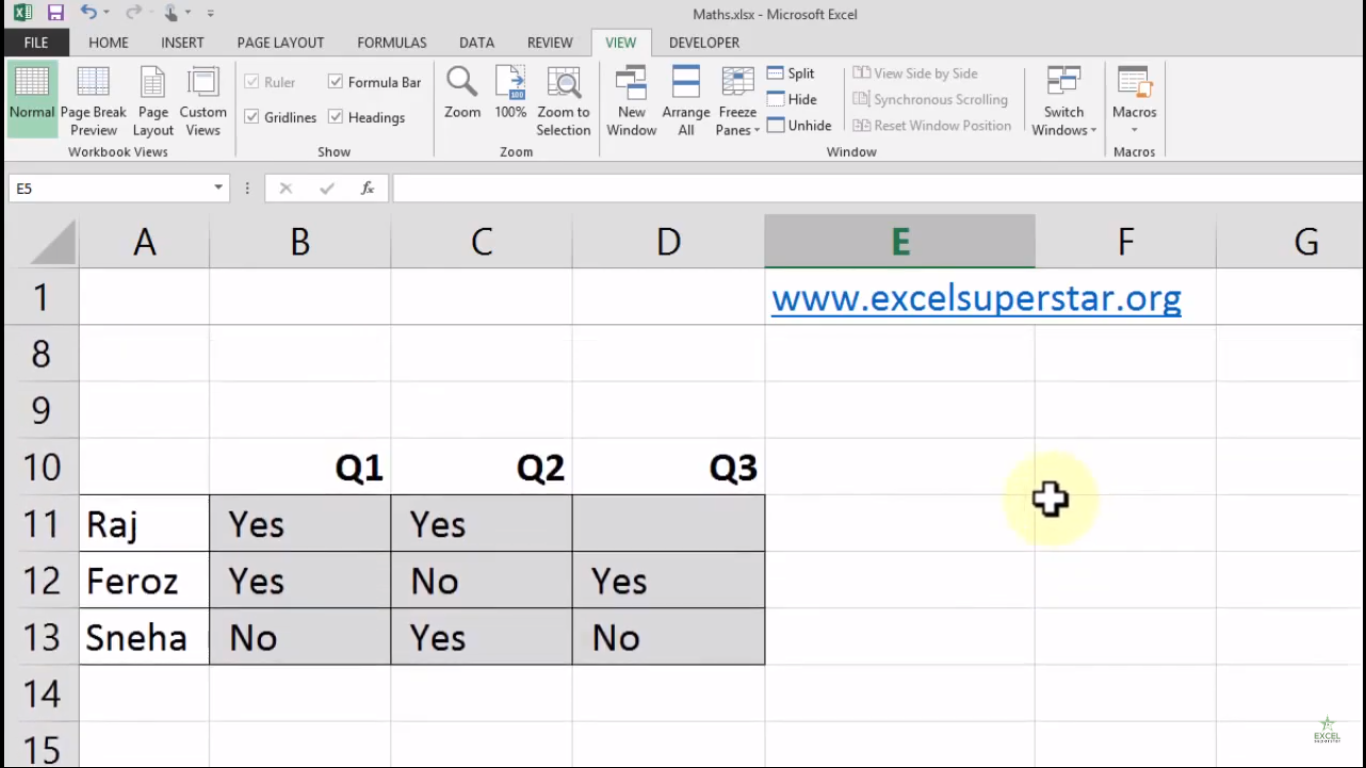2. Select all the grey cells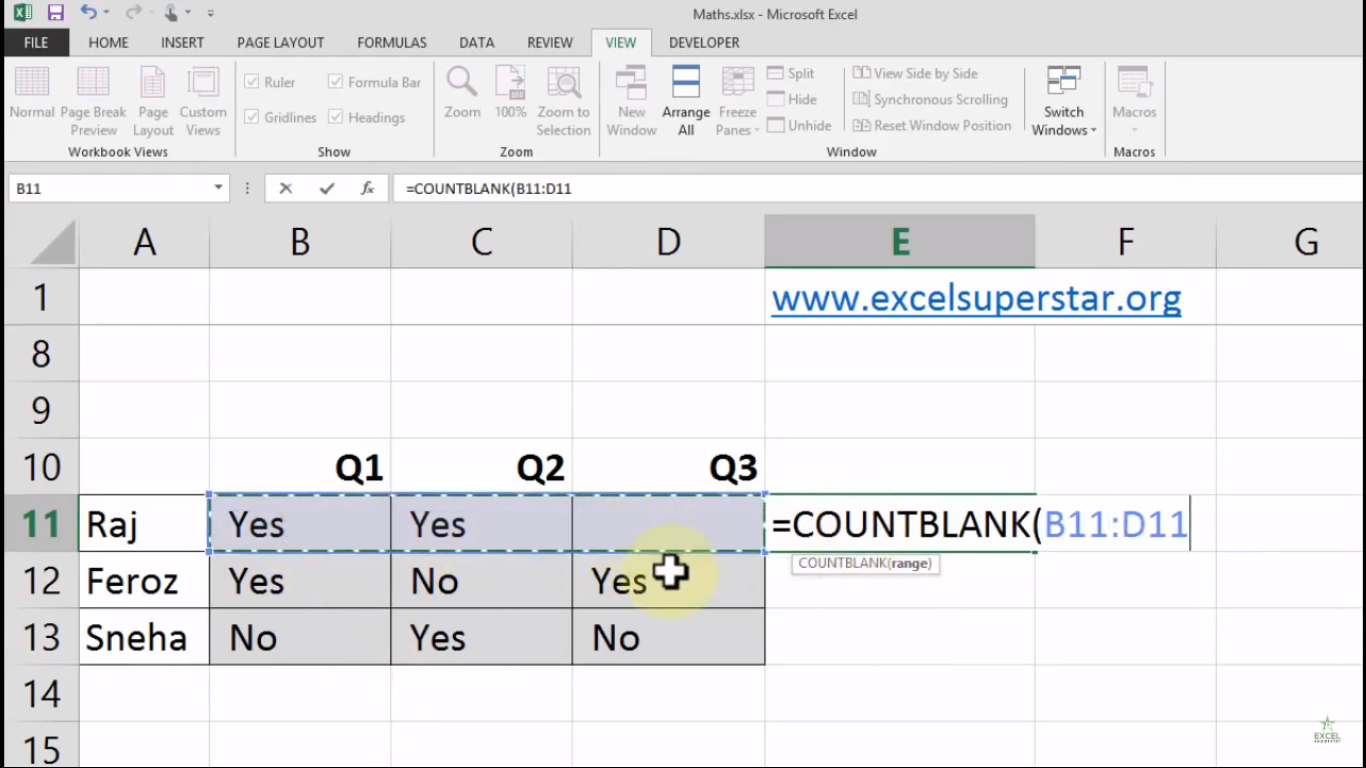3. Close the bracket and press Enter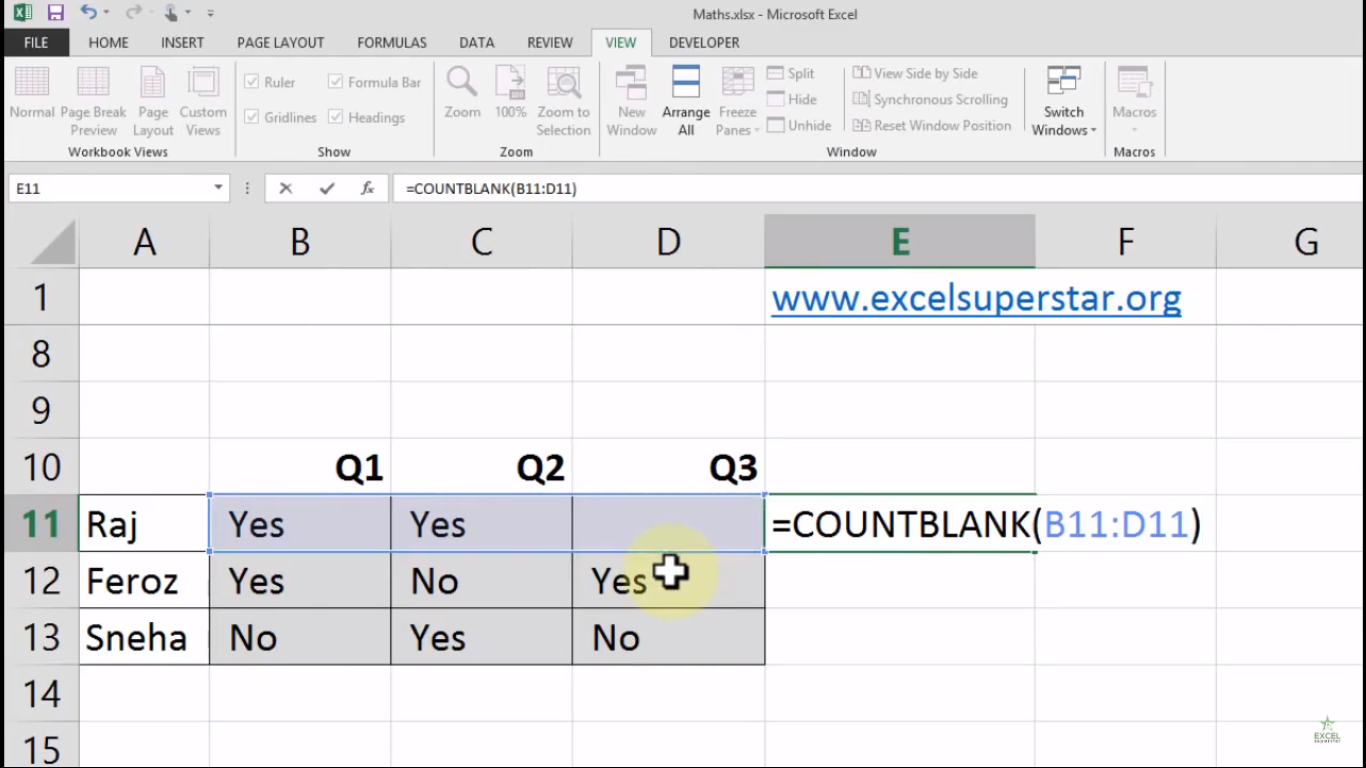4. Lastly, you will get the answer